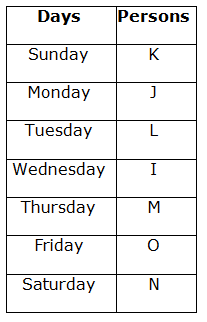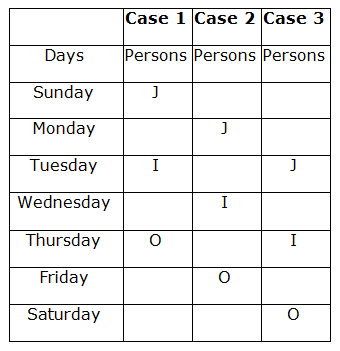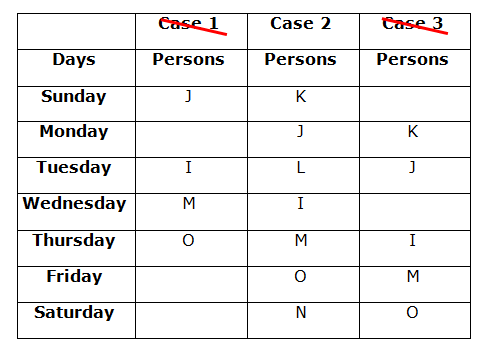# IBPS Clerk Prelims Reasoning Ability Questions 2021 (Day-06)

Dear Aspirants, Our IBPS Guide team is providing new series of Reasoning Questions for IBPS Clerk Prelims 2021 so the aspirants can practice it on a daily basis. These questions are framed by our skilled experts after understanding your needs thoroughly. Aspirants can practice these new series questions daily to familiarize with the exact exam pattern and make your preparation effective.

Start Quiz

Day based puzzle

Direction (1-5): Study the following information carefully and answer the questions given below.

Seven persons I, J, K, L, M, N, and O are going to a food stall on seven different days of a week from Sunday to Saturday.

O goes to a food stall four days after J. As many persons go to a food stall between J and I is same as the persons go to a food stall between O and I. Three persons go to a food stall between K and M, who go to a food stall immediately after I. L goes to a food stall before N, but not goes to a food stall at first.

1) Who among the following persons goes on Saturday?

A.N

B.The persons who go immediately after J

C.The persons who go immediately after O.

D.M

E.Both A & C

2) How many persons go to a food stall between L and N?

A.As many persons go between M and J

B.As many persons go between O and J

C.As many persons go between M and K

D.Both B & C

E.None of these

3) Four of the following five are alike in a certain way and thus form a group as per the given arrangement. Which of the following does not belong to that group?

A.MJ

B.IN

C.KI

D.LO

E.IN

4) Which of the following statements is/are false?

A.L goes on Tuesday

B.N goes to a food stall at last

C.Only two persons go between M and N

D.As many persons go before and after I is same

E.None is false

5) Who among the following goes exactly between J and N?

A.I

B.O

C.L

D.M

E.Both A & D

Inequality

Direction (6-9): In these questions, the relationship between different elements is shown in the statement. The statements are followed by two conclusions. Choose the correct Answer given below:

6) Statements: Z ≥ E < F ≤ O; O ≥ K > M ≥ L

Conclusions:

I) L < O

II) Z > M

A.Only conclusion I is true

B.Only conclusion II is true

C.Either conclusion I or conclusion II is true

D.Both conclusion I and II are true

E.Neither conclusion I nor II is true

7) Statements:
P = A; U ≤ S; A ≥ M > U

Conclusions:

I)P > U

II)S > A

A.Only conclusion I is true

B.Both conclusion I and II are true

C.Either conclusion I or II is true

D.Neither conclusion I nor II is true

E.Only conclusion II is true

8) Statements:
Y < Q; B ≤ E = X < A < Z ≤ Y

Conclusions:

I)B < Y

II)Q > E

A.Only conclusion II is true

B.Only conclusion I is true

C.Both conclusion I and II are true

D.Either conclusion I or II is true

E.Neither conclusion I nor II is true

9) Statements:
R = Q; D ≤ F; Q ≥ T > D

Conclusions:

I)R ≥ D

II)F > Q

A.Only conclusion I is true

B.Only conclusion II is true

C.Both conclusion I and II are true

D.Either conclusion I or II is true

E.Neither conclusion I nor II is true

Miscellaneous

10) If in the number 563821496, the digits at even positions (from the left end) are dropped and the remaining digits are arranged in ascending order from the left end, then which of the following number is fourth from the right end?

A.3

B.5

C.4

D.2

E.6

Directions (1-5) :We have,

• O goes to a food stall four days after J.

As many persons go to a food stall between J and I is the same as the persons go to a food stall between O and I.Again we have,

• Three persons go to a food stall between K and M, who go to a food stall immediately after I.
• L goes to a food stall before N, but not goes to a food stall at first.After arranging the series, we get;

Z ≥ E < F ≤ O ≥ K > M ≥ L

I) L < O (true)

II) Z > M (false)

After arranging the series, we get;

P = A ≥ M > U ≤ S

I) P >U(true)

II) S > A (false)

After arranging the series, we get;

B ≤ E = X < A < Z ≤ Y < Q

I) B <Y(true)

II) Q > E (true)

After arranging the series, we get;

R = Q ≥ T > D ≤ F

I) R ≥ D (false)

II) F > Q (false)